Courses
Courses for Kids
Free study material
Free LIVE classes
More

# The Circle Diagram - Construction, Components and Application in Induction MotorsLIVE
Join Vedantu’s FREE Mastercalss

## A Brief about Induction Motors and Circle Diagrams

Circle diagram is basically just a tool that is used to study the performance of an induction motor under all operating conditions. So before moving onto a circle diagram, it is important to get a basic idea about induction motors if you don’t already know about them. An induction motor is an AC electric motor, and they are also known as asynchronous motors. Through electromagnetic induction, the revolving magnetic field of the stator winding provides the electric current required to generate torque in the rotor. Since the motor works using electromagnetic induction, it is known as an induction motor.

The induction motor has various operating conditions and to keep a hold of all the information is a difficult task at times. The performance of an induction motor is represented graphically using a circle diagram. The circle diagram shows data about the induction motor's power output, losses, and efficiency.

## Solving the Induction Circuit

A circle diagram is based on an approximate equivalent circuit of the induction motor. The diagram is shown below. This diagram is also known as an induction circuit diagram.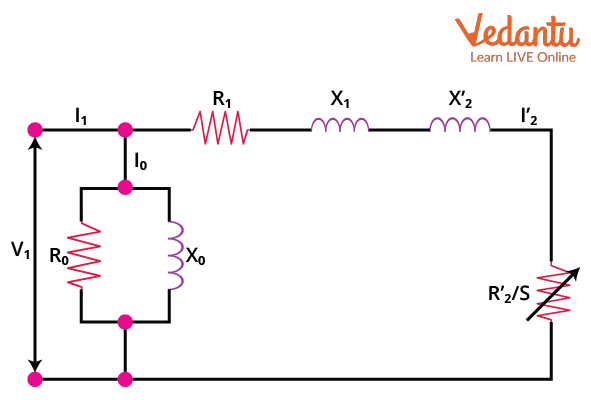Equivalent circuit for circle diagram

Applying Kirchhoff’s current law in the circuit above we get that,

$I_{1}=I_{0}+I_{2}{ }^{\prime}$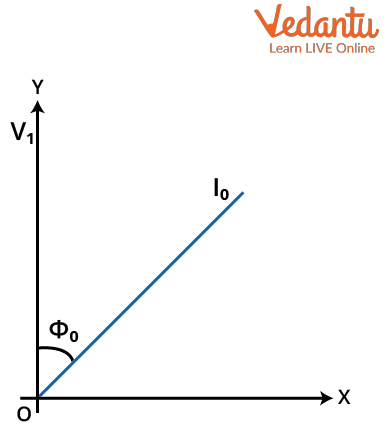Phase diagram between V1 and I0

If we draw a phase diagram between I0 and V1 we find that the no load current I0 lags behind V1 by an angle which we can consider to be $\phi_0$, and it is called no load factor angle. The no load factor angle is between 60 and 80 degrees.

At no load, the slip s is equal to 0. This means that,

$\dfrac{R_{2}}{s}=\infty$

$\dfrac{R_2}{s}$ is an open circuit for the no load condition.

Now we have,

\begin{align} &I_{0}=\dfrac{V_{1}}{Z_{N L}} \\ &Z_{N I .}=\left(R_{0} \| j X_{0}\right) \end{align}

ZNL is the no load impedance of the circuit. All the rotational losses are considered by R0 and the formula for the no loadloss is,

$P_{0}=V_{1} I_{0} \cos \phi_{0}$

The source of the rotor current directed toward the stator side is,

$I_{2}^{\prime}=\dfrac{V_{1}}{\sqrt{\left(R_{1}+\dfrac{R_{2}^{\prime}}{s}\right)^{2}+\left(X_{1}+X_{2}^{\prime}\right)^{2}}}$

This current lags behind the voltage V1. The impedance angle is given by ϕ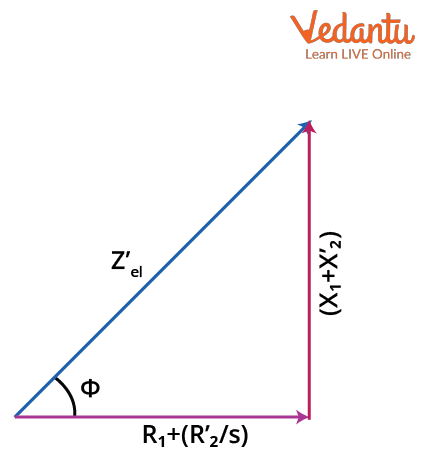Diagram for the impedance angle

From the above diagram we can write,

$\sin \varphi=\dfrac{X_{1}+X_{2}^{\prime}}{\sqrt{\left(R_{1}+\dfrac{R_{2}{ }^{\prime}}{s}\right)^{2}+\left(X_{1}+X_{2}^{\prime}\right)^{2}}}$

By using the equations of sinϕ and I2’ we can write,

$I_{2}^{\prime}=\dfrac{V_{1}}{X_{1}+X_{2}{ }^{\prime}} \sin \varphi$

This equation has a form of $r=a\sin \varphi$. This equation represents the circle that has a diameter a.

We can then say that the locus of I2 is a circle having diameter,

$\dfrac{V_{1}}{X_{1}+X_{2}^{\prime}}$

Using this we can then form a circle diagram as given below.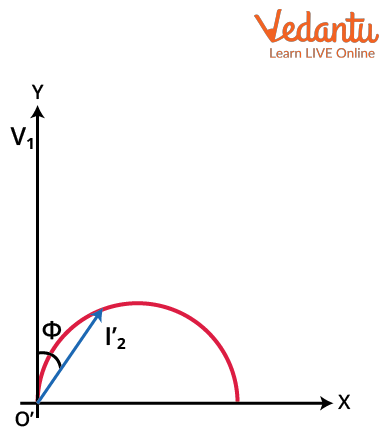Circle diagram of induction circuit

The equation,

$I_{2}^{\prime}=\dfrac{V_{1}}{X_{1}+X_{2}^{\prime}} \sin \varphi$  is known as the periphery of the circle formula because it forms the locus of the current.

## Computing Data for a Circle Diagram

We have the circuit in the section before, but we still need data to construct a circle diagram. The data used is known as the parameter of the circle. This data can be gained by performing tests on the induction motor which can be obtained from:

• Blocked rotor test

• Stator resistance test

The no load test involves a calculation of the total power consumed by the machine,  while the induction motor runs under no load condition at rated power supply. Then observe the supply line voltage represented by V0, no load line current I0 and no load power P0. To calculate the data, two watmeters are used and the slip of the motor is taken to be zero. This test gives us the no load current as well as the angle subtended between the voltage and the current vectors. Owing to the high inductive resistance that the induction motor exhibits under no load conditions, the angle between the current and voltage becomes pretty large. The phase angle for no-load condition

$\phi_0$ is given as,

$\phi_{0}=\dfrac{P_{0}}{\sqrt{3} V_{0} I_{0}}$

## Blocked Rotor Test

The blocked rotor test performed on the induction motor gives us the necessary information to calculate the short circuit current and the angle by which the current lags behind the voltage. This test is similar to that of the short-circuiting of the secondary connections of a transformer. The rotor is blocked firmly, and then a reduced voltage is applied to obtain the rated current at motor terminals. The supply line voltage VSC, no load line current ISC and the no load power PSC are then observed. From this test we can get the phase angle for blocked rotor condition $\phi_{SC}$, current drawn if rated voltage is applied at blocked rotor condition ISN and the power input at rated voltage and motor in the blocked rotor condition PSN. The formulae for these parameters are:

\begin{align} \phi_{S C} &=\dfrac{P_{S C}}{\sqrt{3} V_{S C} I_{S C}} \\ I_{S N} &=I_{S C} \dfrac{V_{0}}{V_{S C}} \\ P_{S N} &=P_{S C}\left(\dfrac{V_{0}}{V_{S C}}\right)^{2} \end{align}

The periphery of the circle is calculated using these formulae, and they are called the periphery of the circle formula.

## Stator Resistance Test

The voltmeter ammeter test is a general method that is used to measure electrical resistance. Simultaneous readings of the current and the voltage are to be taken and then Ohm’s law can be used to find the resistance. So the per phase equivalent stator resistance R1 is to be determined using the voltmeter ammeter method. If the machine is wound rotor type the equivalent rotor resistance R2’ is also required. It can be found after measuring the rotor resistance and then applying the required transformations.

## Construction of the Circle Diagram of an Induction Motor

We now have the required data to plot the circle diagram. Just to mention again the data that we require is:

• Stator phase voltage

• No load power factor $(\cos{\phi_0})$

• Stator resistance per phase R1

• Blocked rotor current ISC

• Blocked rotor power factor PSC

The steps followed to draw a circle diagram are:

• Represent the horizontal axis as OX and the vertical axis as OV. OV is the voltage reference for our diagram.

• With any scale of your choice then draw a phasor represented by OA with a length corresponding to I0. The scale used is called the circle scale. OA is drawn at an angle $\phi_0$ with respect to OV. Then a horizontal line AB is to be drawn from A.

• Then draw another line OS that is equal to ISN. Draw this at an angle equal to $\phi_{SC}$ and join A with S.

• Then draw the perpendicular bisector of AS and extend it such that it meets the horizontal line AB and name the point of intersection as C.

• Then with C as the center, draw a semicircle that passes through A and S. This is actually the circle diagram, and it is the locus of the input current.

• From S, draw a vertical line SL and make it meet AB.

• Now we have to find a point K on the line SL.

• For wound rotor machines the equivalent resistance is R2and K can be found by dividing SL at point K such that

SK:KL=equivalent motor resistance:stator resistance

• For squirrel cage rotor machines the stator copper loss can be found using ISN and stator winding resistance R1. The rotor copper loss is equal to the difference of the total copper loss and stator copper loss.

Then the line SL is to be divided at point K such that,

SK:KL=rotor copper loss:stator copper loss

• After finding K, for any operating point P draws a vertical line PEFGD as shown in the circle diagram below.

The parts of a circle diagram are,

We then have,

• PD=input power

• PE=output power

• EF=rotor copper loss

• FG=stator copper loss

• GD=constant loss

Efficiency of the machine at operating point P=PE/PD

Slip of the machine at operating point P=EF/PD

Starting torque at rated voltage= SK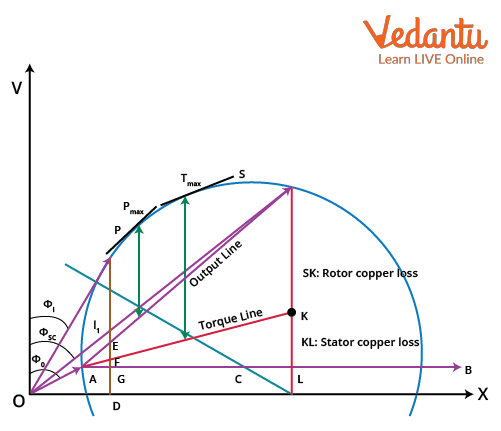Circle Diagram of an induction motor

Drawing tangents to the circle diagram parallel to the output line and torque line will give the operating points corresponding to maximum power and maximum torque respectively. The points where the tangents touch the circle are the maximum power point and the maximum torque points as shown in the diagram.

### Components of a Circle Diagram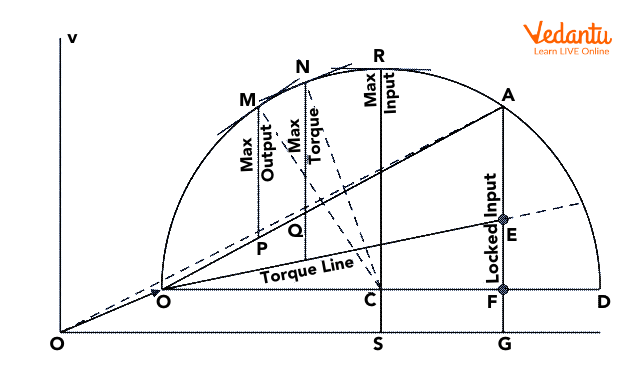Different parts of a circle diagram

A circle diagram has three distinct parts, as we can see in the above image. The three major parts of a circle diagram are the maximum output power, the maximum input power, and the maximum value of torque. We discussed this before, but we’ll see this in detail now.

• Maximum Torque:  Referring to the figure, you can see that when the tangent to the circle is parallel with the torque line. A line from the center O is drawn perpendicular to the torque line OE. This line, when extended further, cuts the circle at point N.

• Maximum Output Power: As you can see from the figure, the maximum output power is obtained when the tangent to the circle is parallel to the red dotted line. The perpendicular dropped from the centre to the output line ends up at the point M where it intersects the circle.

• Maximum Input Power:  The maximum input power is represented by the point R which is the highest point on the periphery of the circle formula. It is obtained when the tangent to the circle is at right angles to the horizontal line.

### Importance of a Circle Diagram

The circle diagram differs from an ordinary phasor diagram in the fact that it provides more information than a phasor diagram. We know that a phasor diagram gives the relation between current and voltage at a particular condition of the circuit. For different conditions, we have different phasor diagrams, and it is cumbersome to draw phasor diagrams for every condition. However, under several circuit circumstances, a circle diagram may be referred to as a phasor diagram shown in a single plane.

As we have already seen that we found the power output, power factor, torque, slip, copper loss, efficiency, input power, etc from the circle diagram, it is not surprising that this diagram is important for induction motors where all these parameters are crucial. A single diagram gives us information about all these parameters.

## Conclusion

An electrical machine is depicted graphically in a circle diagram. It represents the electrical machine's performance and efficiency. The circle scale may depict the same for diverse circuit conditions in a single plane, unlike the phasor diagram, which only offers us the graphical representation of a circuit under specified conditions and changes when these conditions change. The no load test, blocked rotor test and the resistance test need to be performed to get the required parameters for drawing the circle diagram. The three chief parts of the circle diagram are the maximum output power, maximum input power, and the maximum torque produced by the motor.

Last updated date: 02nd Oct 2023
Total views: 3.3k
Views today: 0.03k

## FAQs on The Circle Diagram - Construction, Components and Application in Induction Motors

1. What are the major parts of an induction motor?

The stator, which is the motor's stationary component, transmits a revolving magnetic field to the rotor so that they can interact. The rotor, which is attached to the shaft, is the main part of the motor. The rotor rotates along with the motor shaft, which is fixed inside of it. The shaft protrudes from the motor's outer casing and enables the transmission of rotational power via a connection to an external system. Bearing and casings are also used.

2. What is the importance of Circle Diagrams in JEE?

As I mentioned earlier Circle diagram is an important tool to solve problems. But each yeartends to give one or two questions in the exam, mainly with complex roots or concepts using range and all that. It can happen that you may not find any direct questions in JEE, But it is a very fundamental and equally important concept that will help you solve other problems in JEE. And I can assure you that you have to solve the Circle Diagram at least once, be that direct question or part of the solution.